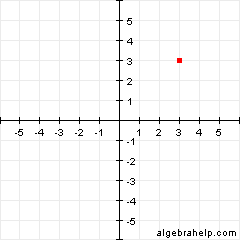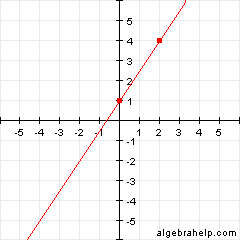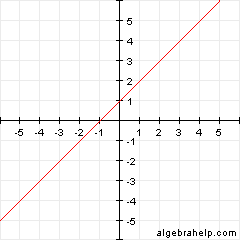# Algebra Help Worksheet List

Below is a list of all our algebra worksheets. Still need help after working through these worksheets? At Wyzant, connect with algebra tutors and math tutors nearby. Prefer to meet online? Find online algebra tutors or online math tutors in a couple of clicks.

## Basics

Basic Equations

 Sample Problem: x + 9 = 18 – 2x Solution: x = 3

Basic Proportions

 Sample Problem: ```4 8 - = -- x 20``` Solution: x = 10

Consecutive Integer Word Problems

 Sample Problem: What are two consecutive even integers that have a sum of 26? Solution: 12, 14

## Simplifying

Simplifying and Solving Equations with Multiple Signs

 Sample Problem: – -16 – x = 13 Solution: x = 3

Simplifying and Solving Equations by Combining Like Terms

 Sample Problem: 6x – 2 + 2x = -2 + 4x + 8 Solution: x = 2

## Multiplication

Two Single Terms

 Sample Problem: 11s * 12s3 Solution: 132s4

Distributive Property

 Sample Problem: 2(4 + 9x) Solution: 8 + 18x

FOIL Method

 Sample Problem: (1 + x)(3 + 2x) Solution: 3 + 5x + 2x2

## Exponents

Of Numbers

 Sample Problem: 52 Solution: 25

Of Variables

 Sample Problem: (k2m3)2 Solution: k4m6

Of Polynomials (Parentheses)

 Sample Problem: (2y + 9)2 Solution: 4y2 + 36y + 81

Order of Operations

 Sample Problem: 3 + 2 * 5x(y + 1) Solution: 3 + 10xy + 10x

## Negative Exponents

Negative Exponents of Numbers

 Sample Problem: 2-3 Solution: 1/8

Negative Exponents of Variables

 Sample Problem: j-3 Solution: 1/j3

Negative Exponents in Fractions

 Sample Problem: a-2b/a Solution: b/a3

## Substitution

Substitution Introduction

 Sample Problem: Evaluate: b + x2, where b = -2 and x = p + 1. Solution: p2 – 2p – 1

## Factoring

Factoring Numbers

 Sample Problem: 15 Solution: 1, 3, 5, 15

Finding a Greatest Common Factor (GCF) of Two Numbers

 Sample Problem: 42, 98 Solution: 14

Finding a Greatest Common Factor (GCF) of Two Terms

 Sample Problem: 21x2y, 7xy3 Solution: 3xy

Factoring a GCF From an Expression

 Sample Problem: 5x2 + 15x3 Solution: 5x2(1 + 3x)

Factoring a Difference Between Two Squares

 Sample Problem: x2 – 49 Solution: (x + 7)(x – 7)

Factoring a Trinomial

 Sample Problem: 5u2 + -11uv + 6v2 Solution: (5u – 6v)(u – v)

Factoring Completely

 Sample Problem: x4 + 7x3 + 12x2 Solution: x2(x + 3)(x + 4)

Solve By Factoring

 Sample Problem: x2 – 12x + 27 = 0 Solution: x = 3, 9

Completing the Square

 Sample Problem: x2 + x – 3.75 = 0 Solution: x = -2.5, 1.5

 Sample Problem: x2 + x – 3.75 = 0 Solution: x = -2.5, 1.5

## Graphing

Reading the Coordinates of Points on a Graph

 Sample Problem:Solution: (3, 3)

Determining the Slope of a Line

 Sample Problem:Solution: slope: 1.5

Determining x and y Intercepts of a Line

 Sample Problem:Solution: y intercept: 1

Determining a Linear Equation From the Graph of a Line

 Sample Problem:Solution: y = x + 1

Determining a Linear Equation From Two Points (Using the two-point formula)

 Sample Problem: (5, 13) and (10, 23) Solution: y = 2x + 3
Scroll to Top